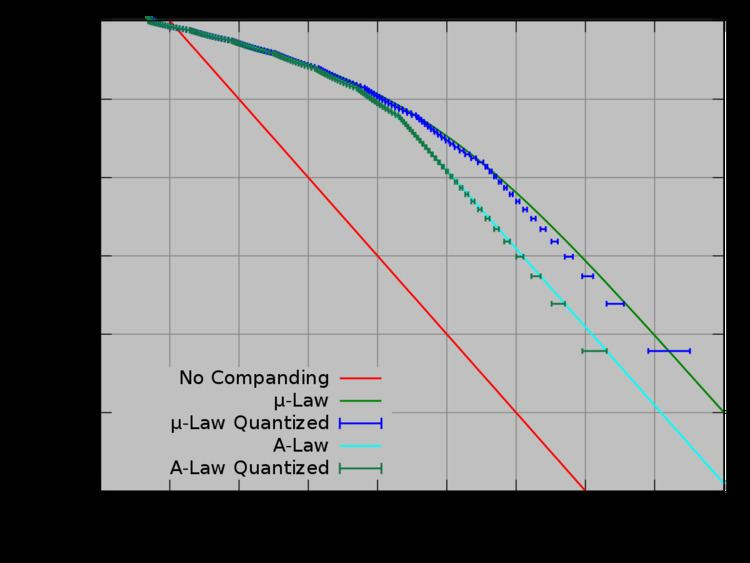# A law algorithm

Updated on
Covid-19An A-law algorithm is a standard companding algorithm, used in European 8-bit PCM digital communications systems to optimize, i.e., modify, the dynamic range of an analog signal for digitizing. It is one of two versions of the G.711 standard from ITU-T, the other version being the similar µ-law, used in North America and Japan.

For a given input x, the equation for A-law encoding is as follows,

F ( x ) = sgn ( x ) { A | x | 1 + log ( A ) , | x | < 1 A 1 + log ( A | x | ) 1 + log ( A ) , 1 A | x | 1 ,

where A is the compression parameter. In Europe, A = 87.6 .

A-law expansion is given by the inverse function,

F 1 ( y ) = sgn ( y ) { | y | ( 1 + ln ( A ) ) A , | y | < 1 1 + ln ( A ) exp ( | y | ( 1 + ln ( A ) ) 1 ) A , 1 1 + ln ( A ) | y | < 1.

The reason for this encoding is that the wide dynamic range of speech does not lend itself well to efficient linear digital encoding. A-law encoding effectively reduces the dynamic range of the signal, thereby increasing the coding efficiency and resulting in a signal-to-distortion ratio that is superior to that obtained by linear encoding for a given number of bits.

## Comparison to μ-law

The μ-law algorithm provides a slightly larger dynamic range than the A-law at the cost of worse proportional distortion for small signals. By convention, A-law is used for an international connection if at least one country uses it.

## References

Similar Topics
Hatters Castle (film)
Tony Atlas
Felicia Fox
Topics